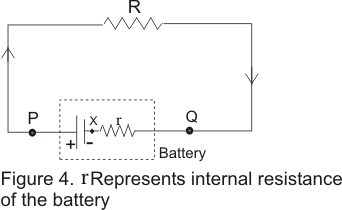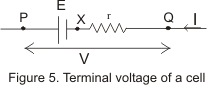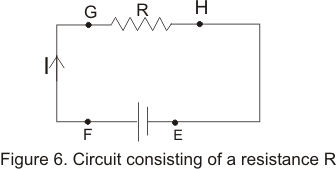# Internal Resistance of Battery|electric energy|Electric power

## (3)Internal Resistance of Battery (or cell)

• The resistance offered by medium in between plates of battery (electrolytes and electrodes of the cell) to the flow of current within the battery is called internal resistance of the battery.
• Internal resistance of a battery usually d branch containing batteryenoted by r and in electric circuit its representation is shown below in the figure• Internal resistance of a battery depends on factors like seperation between plates, plate area, nature of material of plate etc. For an ideal cell r=0 , but real batteries or sourcesof emf always has same finite internal resistance.
• If P and Q are two terminals of the battery shown below in the figurethen potential difference between terminals P and Q is
VP=(VP-Vx) - (VQ - Vx) = E-Ir
let VP-VQ=V
V=E-IR
now for I=0 and V=EMF
and this potential difference V is called the terminal difference of the cell or battery and defined as the emf of the battery when no current drawn from it.
• For real battery equation(4) which gives V=E-Ir whereI is the current in the branch containing battery.
• From figure(4) potential difference across the external resistance R of the circuit would be equal to terminal potential difference of the cell. Thus
V=IR also V=E-Ir
or, IR=E-Ir
which gives
I=E/(R+r) =Net EMF/Net resistance
• From equation(4) we can calculate that when current is drawn from the battery terminal potential difference is less than the EMF of the battery.

## (4)Electric Energy and Power

• To understand the process of energy transfer in a simple circuit consider a simple circuit as shown in the figure given below• Positive terminal of the battery as we all know is always at higher potential.
• Let ΔQ amount of charge begin to flow in the circuit from point E through the battery and resistor and then back to point E.
• When Charge ΔQ moves from point E to point F through the battery electric potential potential energy of the system increased by the amount
ΔU=ΔQ V -(6) and the electric energy of the battery decreased by the same amount.
• When charge ΔQ moves from point G to S through resistoe R, there comes a decrease in electric potential energy.
• This loss in potential energy appears as the increased in thermal energy of the resistor.
• Thermal energy of the resistor increases because when the charge moves through the resistor they loss there electrical potential energy by colliding with the atom in the resistor. This way electrical energy is transformed internal energy crossesponding to increase in vibrational motion of the atom of the resistor and this cause increase in temprature of the resistor.
• The connecting wires are assumed to have negligible resistance and no energy transfer occur for the path FG and HE.
• In time Δt charge ΔQ moves through the resistor i.e. from G to H. The rate at which it loss potential energy
ΔU/Δt=(ΔQ/Δt)ΔV=IΔV where I is thecurrent in the resistor and ΔV is the potential difference across it.
• This charge ΔQ regain its energy when it passes through the battery at the cost of conservation of chemical energy of electrolyte to the electrical energy.
• This loss of potential energy as stated earlier appears as increased thermal energy of the resistor. If P represents the rate at which energy is delivered to the resistor then
P=IΔV
• We know that ΔV =IR for a resistor hence alternative forms of equation(8) are
P=I2R=ΔV2/R where I is expressed in amperes, ΔV iv volts and resustance R in ohm(Ω)
• SI unites of power is watt such that
1watt=1volt*1ampare
Bigger unit of electric power are Kilowatt(KW) and Megawatt(MW).